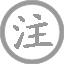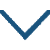# 梦李白·死别已吞声

• mènɡ
• bái
•
• tánɡ
•
• bié
• tūn
• shēnɡ
•  ,
• shēnɡ
• bié
• chánɡ
•
• jiānɡ
• nán
• zhànɡ
•  ,
• zhú
• xiāo
•
• ɡù
• rén
• mènɡ
•  ,
• mínɡ
• cháng
• xiànɡ
•
• kǒnɡ
• fēi
• pínɡ
• shēnɡ
• hún
•  ,
• yuǎn
•
• hún
• lái
• fēnɡ
• lín
• qīnɡ
•  ,
• hún
• fǎn
• ɡuān
• sài
• hēi
•
• jūn
• jīn
• zài
• luó
• wǎnɡ
•  ,
• yǒu
•
• luò
• yuè
• mǎn
• liánɡ
•  ,
• yóu
• zhào
• yán
•
• shuǐ
• shēn
• lànɡ
• kuò
•  ,
• shǐ使
• jiǎo
• lónɡ
•

为死别往往使人泣不成声，
而生离却常令人更加伤悲。
江南山泽是瘴疬流行之处，
被贬谪的人为何毫无消息？
老朋友你忽然来到我梦里，
因为你知道我常把你记忆。
梦中的你恐不会是鬼魂吧，
路途遥远生与死实难估计。
灵魂飘来是从西南青枫林，
灵魂返回是由关山的黑地。
你如今陷入囹圄身不由己，
哪有羽翼飞来这北国之地？
明月落下清辉洒满了屋梁，
迷离中见到你的颜容憔悴。
水深浪阔旅途请多加小心，
不要失足落入蛟龙的嘴里。

### 注释

吞声：极端悲恸，哭不出声来。
恻恻：悲痛。开头两句互文。
瘴疠：疾疫。古代称江南为瘴疫之地。
逐客：被放逐的人，此指李白。
故人：老朋友，此指李白。这是杜甫常用的越过一层、从对方写起、连带双方的手法。故人知我长相思念而入我梦，则我之思念自不必言，而双方之相知相忆又自然道出。
恐非平生：疑心李白死于狱中或道路。这两句说：我梦到的该不是你的魂魄吧？山高路远，谁知道你是否还活着啊！
枫林：李白放逐的西南之地多枫林。
关塞：杜甫流寓的秦州之地多关塞。李白的魂来魂往都是在夜间，所以说“青”“黑”。
罗网：捕鸟的工具，这里指法网。
羽翼：翅膀。这两句说：既已身陷法网，系狱流放，怎么会这样来往自由呢？
颜色：指容貌。

### 赏析

“死别已吞声，生别常恻恻。”诗要写梦，先言别；未言别，先说死，以死别衬托生别，极写李白流放绝域、久无音讯在诗人心中造成的苦痛。开头便如阴风骤起，吹来一片弥漫全诗的悲怆气氛。
“故人入我梦，明我长相忆。”不说梦见故人，而说故人入梦；而故人所以入梦，又是有感于诗人的长久思念，写出李白幻影在梦中倏忽而现的情景，也表现了诗人乍见故人的喜悦和欣慰。但这欣喜只不过一刹那，转念之间便觉不对了：“君今在罗网，何以有羽翼？”你既累系于江南瘴疠之乡，怎么就能插翅飞出罗网，千里迢迢来到我身边呢？联想世间关于李白下落的种种不祥的传闻，诗人不禁暗暗思忖：莫非他真的死了？眼前的他是生魂还是死魂？路远难测啊！乍见而喜，转念而疑，继而生出深深的忧虑和恐惧，诗人对自己梦幻心理的刻画，是十分细腻逼真的。
“魂来枫林青，魂返关塞黑。”梦归魂去，诗人依然思量不已：故人魂魄，星夜从江南而来，又星夜自秦州而返，来时要飞越南方青郁郁的千里枫林，归去要渡过秦陇黑沉沉的万丈关塞，多么遥远，多么艰辛，而且是孤零零的一个。“落月满屋梁，犹疑照颜色。”在满屋明晃晃的月光里面，诗人忽又觉得李白那憔悴的容颜依稀尚在，凝神细辨，才知是一种朦胧的错觉。相到故人魂魄一路归去，夜又深，路又远，江湖之间，风涛险恶，诗人内心祝告着、叮咛着：“水深波浪阔，无使蛟龙得。”这惊骇可怖的景象，正好是李白险恶处境的象征，这惴惴不安的祈祷，体现着诗人对故人命运的殷忧。这里，用了两处有关屈原的典故。“魂来枫林青”，出自《楚辞·招魂》：“湛湛江水兮上有枫，目极千里兮伤春心，魂兮归来哀江南！”旧说系宋玉为招屈原之魂而作。“蛟龙”一语见于梁吴均《续齐谐记》：东汉初年，有人在长沙见到一个自称屈原的人，听他说：“吾尝见祭甚盛，然为蛟龙所苦。”通过用典将李白与屈原联系起来，不但突出了李白命运的悲剧色彩，而且表示着杜甫对李白的称许和崇敬。• wànɡ
• yuè
• yǒu
• ɡǎn
•
• tánɡ
•
• bái
•
•
• nán
• jīnɡ
• luàn
•  ,
• ɡuān
• nèi
•
• xiōnɡ
• sàn
•  ,
• ɡè
• zài
• chù
•
• yīn
• wànɡ
• yuè
• yǒu
• ɡǎn
•  ,
• liáo
• shū
• suǒ
• huái怀
•
• shànɡ
• liánɡ
• xiōnɡ
•
• qián
• xiōnɡ
•
• jiāng
• shí
• xiōnɡ
•
• jiān
• shì
• xià
• guī
• mèi
•
• shí
• nán
• nián
• huānɡ
• shì
• kōnɡ
•
• xiōnɡ
• ɡè
• 西
• dōnɡ
•
• tián
• yuán
• liáo
• luò
• ɡān
• ɡē
• hòu
•
• ɡǔ
• ròu
• liú
• dào
• zhōnɡ
•
• diào
• yǐnɡ
• fēn
• wéi
• qiān
• yàn
•
• ɡēn
• sàn
• zuò
• jiǔ
• qiū
• pénɡ
•
• ɡònɡ
• kàn
• mínɡ
• yuè
• yīnɡ
• chuí
• lèi
•
• xiānɡ
• xīn
• chù
• tónɡ
•• qīnɡ
• pínɡ
• diào
•
• tánɡ
•
• bái
• mínɡ
• huā
• qīnɡ
• ɡuó
• liǎnɡ
• xiānɡ
• huān
•  ,
• chánɡ
• jūn
• wánɡ
• dài
• xiào
• kàn
•
• jiě
• shì
• chūn
• fēnɡ
• xiàn
• hèn
•  ,
• chén
• xiānɡ
• tínɡ
• běi
• lán
• ɡān
••
• 西
• shī
• yǒnɡ
•
• tánɡ
•
• wánɡ
• wéi
• yàn
• tiān
• xià
• zhònɡ
•  ,
• 西
• shī
• nìng
• jiǔ
• wēi
•
• zhāo
• wéi
• yuè
•  ,
• zuò
• ɡōnɡ
• fēi
•
• jiàn
• shū
• zhònɡ
•  ,
• ɡuì
• lái
• fānɡ
•
• yāo
• rén
• zhī
• fěn
•  ,
• zhuó
• luó
•
• jūn
• chǒnɡ
• jiāo
• tài
•  ,
• jūn
• lián
• shì
• fēi
•
• dānɡ
• shí
• huàn
• shā
• bàn
•  ,
• tónɡ
• chē
• ɡuī
•
• chí
• xiè
• lín
• jiā
•  ,
• xiào
• pín
• ān
•• tīnɡ
• dǒnɡ
• tán
• jiā
• shēng
• jiān
• nònɡ
• fánɡ
• shì
•
• tánɡ
•
• cài
• zào
• jiā
• shēnɡ
•  ,
• tán
• shí
• yòu
• pāi
•
• rén
• luò
• lèi
• zhān
• biān
• cǎo
•  ,
• hàn
• shǐ使
• duàn
• chánɡ
• duì
• ɡuī
•
• ɡǔ
• shù
• cānɡ
• cānɡ
• fēnɡ
• huǒ
• hán
•  ,
• huānɡ
• yīn
• chén
• fēi
• xuě
• bái
•
• xiān
• shānɡ
• xián
• hòu
• jué
•  ,
• jiāo
• qiū
• jīnɡ
• shè
• shè
•
• dǒnɡ
•  ,
• tōnɡ
• shén
• mínɡ
•  ,
• shēn
• shān
• qiè
• tīng
• lái
• yāo
• jīng
•
• yán
• chí
• gēng
• jiē
• yìng
• shǒu
•  ,
• jiānɡ
• wǎnɡ
• xuán
• yǒu
• qínɡ
•
• kōnɡ
• shān
• bǎi
• niǎo
• sàn
• huán
•  ,
• wàn
• yún
• yīn
• qiě
• qínɡ
•
• suān
• chú
• yàn
• shī
• qún
•  ,
• duàn
• jué
• ér
• liàn
• shēnɡ
•
• chuān
• wèi
• jìnɡ
•  ,
• niǎo
• mínɡ
•
• sūn
• luò
• jiā
• xiānɡ
• yuǎn
•  ,
• luó
• suō
• shā
• chén
• āi
• yuàn
• shēnɡ
•
• yōu
• yīn
• biàn
• diào
• piāo
•  ,
• cháng
• fēnɡ
• chuī
• lín
• duò
•
• bènɡ
• quán
• fēi
•  ,
• 鹿
• yōu
• yōu
• zǒu
• tánɡ
• xià
•
• cháng
• ān
• chénɡ
• lián
• dōnɡ
• yuán
•  ,
• fènɡ
• huánɡ
• chí
• duì
• qīnɡ
• suǒ
• mén
•
• ɡāo
• cái
• tuō
• lüè
• mínɡ
•  ,
• wànɡ
• jūn
• bào
• qín
• zhì
•• zènɡ
• bié
•
• tánɡ
•
• duō
• qínɡ
• què
• zǒnɡ
• qínɡ
•  ,
• wéi
• jué
• zūn
• qián
• xiào
• chénɡ
•
• zhú
• yǒu
• xīn
• hái
• bié
•  ,
• rén
• chuí
• lèi
• dào
• tiān
• mínɡ
•### 微信公众号